Open In App

# Find the Factorial of a large number

Find the factorial of a large number.

## What is Factorial of a number?

Factorial of a non-negative integer, is the multiplication of all integers smaller than or equal to n.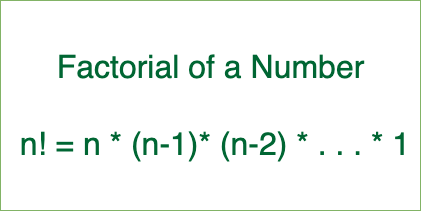Factorial of a number

Examples:

Input: 100
Output: 933262154439441526816992388562667004-
907159682643816214685929638952175999-
932299156089414639761565182862536979-
208272237582511852109168640000000000-
00000000000000

Input: 50
Output: 3041409320171337804361260816606476884-
4377641568960512000000000000

We have discussed a simple program for factorial.

## Why conventional way of computing factorial fails for large numbers?

A factorial of 100 has 158 digits. It is not possible to store these many digits even if we use long int.

The idea is to use basic mathematics for multiplication.

Illustration:

Example to show working of multiply(res[], x)

• A number 5189 is stored in res[] as following: res[] = {9, 8, 1, 5}
• let x = 10
Initialize carry = 0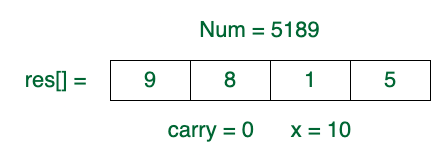• At i = 0, prod = res*x + carry = 9*10 + 0 = 90.
res = 0, carry = 9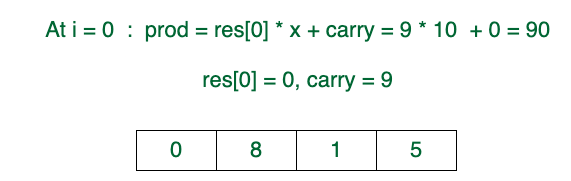• At i = 1, prod = res*x + carry = 8*10 + 9 = 89
res = 9, carry = 8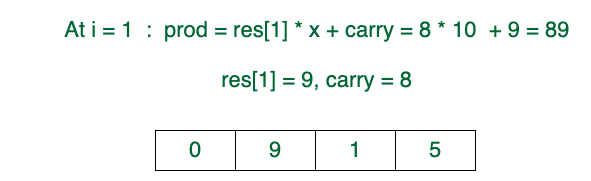• At i = 2, prod = res*x + carry = 1*10 + 8 = 18
res = 8, carry = 1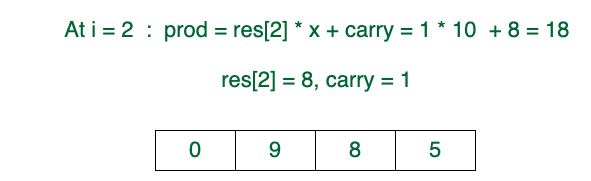• At i = 3, prod = res*x + carry = 5*10 + 1 = 51
res = 1, carry = 5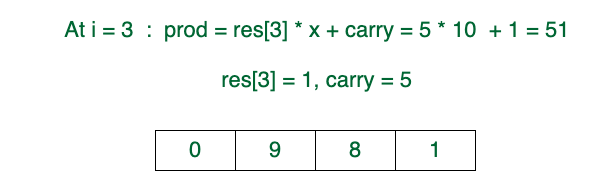• res = carry = 5
res[] = {0, 9, 8, 1, 5}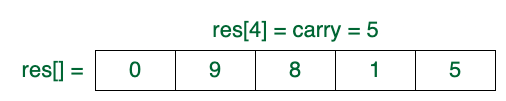Follow the steps below to solve the given problem:

• Create an array res[] of MAX size where MAX is a number of maximum digits in output.
• Initialize value stored in res[] as 1 and initialize res_size (size of ‘res[]’) as 1.
• Multiply x with res[] and update res[] and res_size to store the multiplication result for all the numbers from x = 2 to n.
• To multiply a number x with the number stored in res[], one by one multiply x with every digit of res[].
• To implement multiply function perform the following steps:
• Initialize carry as 0.
• Do following for i = 0 to res_size – 1
• Find value of res[i] * x + carry. Let this value be prod.
• Update res[i] by storing the last digit of prod in it.
• Update carry by storing the remaining digits in carry.
• Put all digits of carry in res[] and increase res_size by the number of digits in carry.

Below is the implementation of the above algorithm.

NOTE: In the below implementation, the maximum digits in the output are assumed as 500. To find a factorial of a much larger number ( > 254), increase the size of an array or increase the value of MAX. This can also be solved using Linked List instead of using res[] array which will not waste extra space.

## C++

 `// C++ program to compute factorial of big numbers``#include ``using` `namespace` `std;` `// Maximum number of digits in output``#define MAX 500` `int` `multiply(``int` `x, ``int` `res[], ``int` `res_size);` `// This function finds factorial of large numbers``// and prints them``void` `factorial(``int` `n)``{``    ``int` `res[MAX];` `    ``// Initialize result``    ``res = 1;``    ``int` `res_size = 1;` `    ``// Apply simple factorial formula n! = 1 * 2 * 3``    ``// * 4...*n``    ``for` `(``int` `x = 2; x <= n; x++)``        ``res_size = multiply(x, res, res_size);` `    ``cout << ``"Factorial of given number is \n"``;``    ``for` `(``int` `i = res_size - 1; i >= 0; i--)``        ``cout << res[i];``}` `// This function multiplies x with the number``// represented by res[].``// res_size is size of res[] or number of digits in the``// number represented by res[]. This function uses simple``// school mathematics for multiplication.``// This function returns the``// new value of res_size``int` `multiply(``int` `x, ``int` `res[], ``int` `res_size)``{``    ``int` `carry = 0; ``// Initialize carry` `    ``// One by one multiply n with individual digits of res[]``    ``for` `(``int` `i = 0; i < res_size; i++) {``        ``int` `prod = res[i] * x + carry;` `        ``// Store last digit of 'prod' in res[]``        ``res[i] = prod % 10;` `        ``// Put rest in carry``        ``carry = prod / 10;``    ``}` `    ``// Put carry in res and increase result size``    ``while` `(carry) {``        ``res[res_size] = carry % 10;``        ``carry = carry / 10;``        ``res_size++;``    ``}``    ``return` `res_size;``}` `// Driver program``int` `main()``{``    ``factorial(100);``    ``return` `0;``}`

## Java

 `// JAVA program to compute factorial``// of big numbers``class` `GFG {` `    ``// This function finds factorial of``    ``// large numbers and prints them``    ``static` `void` `factorial(``int` `n)``    ``{``        ``int` `res[] = ``new` `int``[``500``];` `        ``// Initialize result``        ``res[``0``] = ``1``;``        ``int` `res_size = ``1``;` `        ``// Apply simple factorial formula``        ``// n! = 1 * 2 * 3 * 4...*n``        ``for` `(``int` `x = ``2``; x <= n; x++)``            ``res_size = multiply(x, res, res_size);` `        ``System.out.println(``"Factorial of given number is "``);``        ``for` `(``int` `i = res_size - ``1``; i >= ``0``; i--)``            ``System.out.print(res[i]);``    ``}` `    ``// This function multiplies x with the number``    ``// represented by res[]. res_size is size of res[] or``    ``// number of digits in the number represented by res[].``    ``// This function uses simple school mathematics for``    ``// multiplication. This function may value of res_size``    ``// and returns the new value of res_size``    ``static` `int` `multiply(``int` `x, ``int` `res[], ``int` `res_size)``    ``{``        ``int` `carry = ``0``; ``// Initialize carry` `        ``// One by one multiply n with individual``        ``// digits of res[]``        ``for` `(``int` `i = ``0``; i < res_size; i++) {``            ``int` `prod = res[i] * x + carry;``            ``res[i] = prod % ``10``; ``// Store last digit of``                                ``// 'prod' in res[]``            ``carry = prod / ``10``; ``// Put rest in carry``        ``}` `        ``// Put carry in res and increase result size``        ``while` `(carry != ``0``) {``            ``res[res_size] = carry % ``10``;``            ``carry = carry / ``10``;``            ``res_size++;``        ``}``        ``return` `res_size;``    ``}` `    ``// Driver program``    ``public` `static` `void` `main(String args[])``    ``{``        ``factorial(``100``);``    ``}``}``// This code is contributed by Nikita Tiwari`

## Python3

 `# Python program to compute factorial``# of big numbers` `import` `sys` `# This function finds factorial of large``# numbers and prints them`  `def` `factorial(n):``    ``res ``=` `[``None``]``*``500``    ``# Initialize result``    ``res[``0``] ``=` `1``    ``res_size ``=` `1` `    ``# Apply simple factorial formula``    ``# n! = 1 * 2 * 3 * 4...*n``    ``x ``=` `2``    ``while` `x <``=` `n:``        ``res_size ``=` `multiply(x, res, res_size)``        ``x ``=` `x ``+` `1` `    ``print``(``"Factorial of given number is"``)``    ``i ``=` `res_size``-``1``    ``while` `i >``=` `0``:``        ``sys.stdout.write(``str``(res[i]))``        ``sys.stdout.flush()``        ``i ``=` `i ``-` `1`  `# This function multiplies x with the number``# represented by res[]. res_size is size of res[]``# or number of digits in the number represented``# by res[]. This function uses simple school``# mathematics for multiplication. This function``# may value of res_size and returns the new value``# of res_size``def` `multiply(x, res, res_size):` `    ``carry ``=` `0`  `# Initialize carry` `    ``# One by one multiply n with individual``    ``# digits of res[]``    ``i ``=` `0``    ``while` `i < res_size:``        ``prod ``=` `res[i] ``*` `x ``+` `carry``        ``res[i] ``=` `prod ``%` `10`  `# Store last digit of``        ``# 'prod' in res[]``        ``# make sure floor division is used``        ``carry ``=` `prod``/``/``10`  `# Put rest in carry``        ``i ``=` `i ``+` `1` `    ``# Put carry in res and increase result size``    ``while` `(carry):``        ``res[res_size] ``=` `carry ``%` `10``        ``# make sure floor division is used``        ``# to avoid floating value``        ``carry ``=` `carry ``/``/` `10``        ``res_size ``=` `res_size ``+` `1` `    ``return` `res_size`  `# Driver program``factorial(``100``)` `# This code is contributed by Nikita Tiwari.`

## C#

 `// C# program to compute``// factorial of big numbers``using` `System;` `class` `GFG {` `    ``// This function finds factorial``    ``// of large numbers and prints them``    ``static` `void` `factorial(``int` `n)``    ``{``        ``int``[] res = ``new` `int``;` `        ``// Initialize result``        ``res = 1;``        ``int` `res_size = 1;` `        ``// Apply simple factorial formula``        ``// n! = 1 * 2 * 3 * 4...*n``        ``for` `(``int` `x = 2; x <= n; x++)``            ``res_size = multiply(x, res, res_size);` `        ``Console.WriteLine(``"Factorial of "``                          ``+ ``"given number is "``);``        ``for` `(``int` `i = res_size - 1; i >= 0; i--)``            ``Console.Write(res[i]);``    ``}` `    ``// This function multiplies x``    ``// with the number represented``    ``// by res[]. res_size is size``    ``// of res[] or number of digits``    ``// in the number represented by``    ``// res[]. This function uses``    ``// simple school mathematics for``    ``// multiplication. This function``    ``// may value of res_size and``    ``// returns the new value of res_size``    ``static` `int` `multiply(``int` `x, ``int``[] res, ``int` `res_size)``    ``{``        ``int` `carry = 0; ``// Initialize carry` `        ``// One by one multiply n with``        ``// individual digits of res[]``        ``for` `(``int` `i = 0; i < res_size; i++) {``            ``int` `prod = res[i] * x + carry;``            ``res[i] = prod % 10; ``// Store last digit of``                                ``// 'prod' in res[]``            ``carry = prod / 10; ``// Put rest in carry``        ``}` `        ``// Put carry in res and``        ``// increase result size``        ``while` `(carry != 0) {``            ``res[res_size] = carry % 10;``            ``carry = carry / 10;``            ``res_size++;``        ``}``        ``return` `res_size;``    ``}` `    ``// Driver Code``    ``static` `public` `void` `Main() { factorial(100); }``}` `// This code is contributed by ajit`

## Javascript

 ``

## PHP

 `= 0; ``\$i``--)``        ``echo` `\$res``[``\$i``];``}` `// This function multiplies x with the number``// represented by res[].``// res_size is size of res[] or number of``// digits in the number represented by res[].``// This function uses simple school mathematics``// for multiplication. This function may value ``// of res_size and returns the new value of res_size``function` `multiply(``\$x``, &``\$res``, ``\$res_size``)``{``    ``\$carry` `= 0; ``// Initialize carry` `    ``// One by one multiply n with individual``    ``// digits of res[]``    ``for` `(``\$i` `= 0; ``\$i` `< ``\$res_size``; ``\$i``++)``    ``{``        ``\$prod` `= ``\$res``[``\$i``] * ``\$x` `+ ``\$carry``;` `        ``// Store last digit of 'prod' in res[]``        ``\$res``[``\$i``] = ``\$prod` `% 10;` `        ``// Put rest in carry``        ``\$carry` `= (int)(``\$prod` `/ 10);``    ``}` `    ``// Put carry in res and increase``    ``// result size``    ``while` `(``\$carry``)``    ``{``        ``\$res``[``\$res_size``] = ``\$carry` `% 10;``        ``\$carry` `= (int)(``\$carry` `/ 10);``        ``\$res_size``++;``    ``}``    ``return` `\$res_size``;``}` `// Driver Code``factorial(100);``    ` `// This code is contributed by chandan_jnu``?>`

Output

```Factorial of given number is
93326215443944152681699238856266700490715968264381621468592963895217599993229915608941463976156518286253697920827223758251185210916864000000000000000000000000```

Time Complexity: O(N log (N!)), where O(N) is for loop and O(log N!) is for nested while loop
Auxiliary Space: O(max(digits in factorial))

## Find the Factorial of a large number using Basic BigInteger

This problem can be solved using the below idea:

Big Integer can also be used to calculate the factorial of large numbers.

Illustration:

N = 5

ans = 1

At i = 2: ans = ans x i = 1 x 2 = 2
At i = 3: ans = ans x i = 2 x 3 = 6
At i = 4: ans = ans x i = 6 x 4 = 24
At i = 5: ans = ans x i = 24 x 5 = 120

Hence factorial of N is 120

Follow the steps below to solve the given problem:

• Declare a BigInteger f with 1 and perform the conventional way of calculating factorial
• Traverse a loop from x = 2 to N and multiply x with f and store the resultant value in f

Below is the implementation of the above idea :

## C++

 `// C++ program to find large``// factorials using BigInteger``#include ``using` `namespace` `std;` `#define ull unsigned long long` `// Returns Factorial of N``ull factorial(``int` `N)``{` `    ``// Initialize result``    ``ull f = 1; ``// Or BigInt 1` `    ``// Multiply f with 2, 3, ...N``    ``for` `(ull i = 2; i <= N; i++)``        ``f *= i;` `    ``return` `f;``}` `// Driver method``int` `main()``{``    ``int` `N = 20;``    ``cout << factorial(N) << endl;``}` `// This code is contributed by phasing17`

## Java

 `// Java program to find large``// factorials using BigInteger``import` `java.math.BigInteger;``import` `java.util.Scanner;` `public` `class` `Example {` `    ``// Returns Factorial of N``    ``static` `BigInteger factorial(``int` `N)``    ``{``        ``// Initialize result``        ``BigInteger f``            ``= ``new` `BigInteger(``"1"``); ``// Or BigInteger.ONE` `        ``// Multiply f with 2, 3, ...N``        ``for` `(``int` `i = ``2``; i <= N; i++)``            ``f = f.multiply(BigInteger.valueOf(i));` `        ``return` `f;``    ``}` `    ``// Driver method``    ``public` `static` `void` `main(String args[]) ``throws` `Exception``    ``{``        ``int` `N = ``20``;``        ``System.out.println(factorial(N));``    ``}``}`

## Python3

 `# Python3 program to find large``# factorials` `# Returns Factorial of N``def` `factorial(N):` `    ``# Initialize result``    ``f ``=`  `1` `    ` `    ``# Multiply f with 2, 3, ...N``    ``for` `i ``in` `range``(``2``, N ``+` `1``):``        ``f ``*``=` `i``    ` `    ``return` `f;`  `# Driver method``N ``=` `20``;``print``(factorial(N));`  `# This code is contributed by phasing17`

## C#

 `// C# program to find large``// factorials using BigInteger``using` `System;``using` `System.Collections.Generic;``using` `System.Numerics;` `public` `class` `Example {` `  ``// Returns Factorial of N``  ``static` `BigInteger factorial(``int` `N)``  ``{` `    ``// Initialize result``    ``BigInteger f``      ``= ``new` `BigInteger(1); ``// Or BigInteger.ONE` `    ``// Multiply f with 2, 3, ...N``    ``for` `(``int` `i = 2; i <= N; i++)``      ``f = BigInteger.Multiply(f, ``new` `BigInteger(i));` `    ``return` `f;``  ``}` `  ``// Driver method``  ``public` `static` `void` `Main(``string``[] args)``  ``{``    ``int` `N = 20;``    ``Console.WriteLine(factorial(N));``  ``}``}` `// This code is contributed by phasing17`

## Javascript

 `// JavaScript program to find large``// factorials using BigInteger` `// Returns Factorial of N``function` `factorial(N)``{` `// Initialize result``let f =  BigInt(1); ``// Or BigInt 1` `// Multiply f with 2, 3, ...N``for` `(``var` `i = 2; i <= N; i++)``    ``f *= BigInt(i);` `return` `f;``}` `// Driver method``let N = 20;``console.log(factorial(N));` `// This code is contributed by phasing17`

Output

```2432902008176640000
```

Time Complexity: O(N)
Auxiliary Space: O(1)

## Find Factorial of a number using Linked List :-

So the basic idea is to multiply the next number ranging between 2 to N with the data stored in the current Node and also maintain a carry just like the array approach. And then move on to the next node.

Let’s break it down into following steps :

1. Initially we’ll create 1 single node containing 1 in it.
2. Then initialized i of a for loop with 2.
3. And for each value of i up till N, we’ll call a function multiply which takes 2 parameter, head of the list and value of i.
4. And perform the below operation (See the image)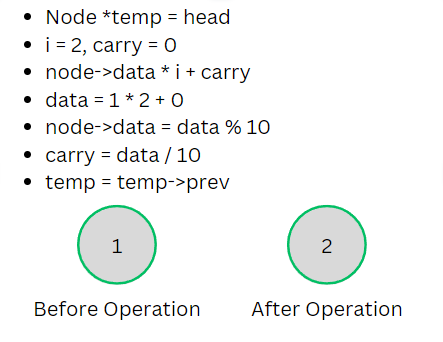Operation

The above operation will be carried out till our temp pointer becomes NULL.

Now there are further 2 cases , The multiplication of i with the node’s data :

• Doesn’t exceed 1 digit
• Exceeds 1 digit

If the multiplication of current node’s data with i – Exceeds 1 digit, then we won’t be storing it into a single node. Rather, we’ll be storing each digit into a single node. And if it doesn’t then we’ll simply replace current node’s data with it.

So, by above explanation we can conclude and form the following steps to solve this problem :

### Algorithm:

1. Find value of : node’s data * i + carry. Store it in a variable ‘prod’.
2. Initialize 2 pointers let’s say prev and temp on the current node and Update the current node’s value by storing the last digit of the ‘prod’
3. Update carry by storing the remaining digits in carry (excluding the last digit)
4. Now, bring prev ptr on temp and move temp to the next node (if any) and then again perform, the previous steps until the carry becomes 0 or the temp ptr becomes NULL.
5. Once the temp pointer reaches the NULL there is a possibility that carry is still not 0. So, until carry becomes 0 we have to again perform the same steps by creating a new node for every remaining digit.

See the image below for the Dry run of above steps :

(i) Node’s data = 1, i = 2, carry = 0.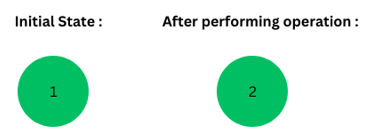(ii) Node’s Data : 2, i = 3, carry = 0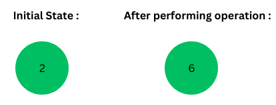(iii) Node’s Data : 6, i = 4, carry = 2 (6*4 = 24, 24 % 10 = 4, 24/10 = 2)

X = NULL in the below image :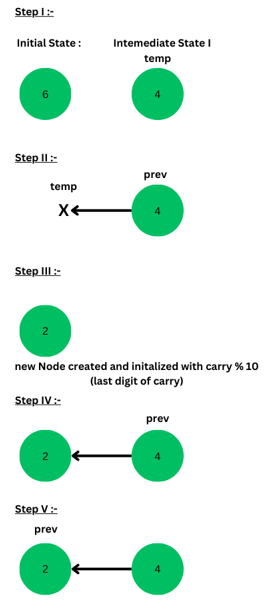6 × 4 = 24

Just like this, we’ll do for the remaining digits, and each of the digit will be stored in a single node.

Code :

## C++

 `#include ``using` `namespace` `std;` `//* Node Class``class` `Node {``public``:``    ``int` `data;``    ``Node* prev;``    ``Node(``int` `n)``    ``{``        ``data = n;``        ``prev = NULL;``    ``}``};` `//* Function to perform desired operation``void` `Multiply(Node* head, ``int` `i)``{``    ``Node *temp = head,``        ``*prevPtr = head; ``// Temp variable for keeping head``    ` `    ``int` `carry = 0;` `    ``//* Perform operation until temp becomes NULL``    ``while` `(temp != NULL) {``        ``int` `prod = temp->data * i + carry;``        ``temp->data = prod % 10; ``//* Stores the last digit``        ``carry = prod / 10;``        ``prevPtr = temp; ``//* Change Links``        ``temp = temp->prev; ``//* Moving temp to next node``    ``}` `    ``//* If carry is greater than 0 then we create new nodes``    ``//* to store remaining digits.``    ``while` `(carry != 0) {``        ``prevPtr->prev = ``new` `Node((``int``)(carry % 10));``        ``carry /= 10;``        ``prevPtr = prevPtr->prev;``    ``}``}` `//* Using head recursion to print the linked list's data in reverse``void` `print(Node* head)``{``    ``if` `(head == NULL)``        ``return``;``    ``print(head->prev);``    ``cout << head->data; ``// Print linked list in reverse order``}` `// Driver code``int` `main()``{``    ``int` `n = 100;``    ``Node *head = ``new` `Node(1); ``// Create a node and initialise it by 1``    ` `    ``for``(``int` `i = 2; i <= n; i++)``        ``Multiply(head, i); ``// Run a loop from 2 to n and``                            ``// multiply with head's i``    ``cout << ``"Factorial of "` `<< n << ``" is : \n"``;``    ``print(head); ``// Print the linked list``    ``cout << endl;``    ``return` `0;``}`

Output

```Factorial of 100 is :
93326215443944152681699238856266700490715968264381621468592963895217599993229915608941463976156518286253697920827223758251185210916864000000000000000000000000
```

Time Complexity : O(N²)
Space Complexity : O(digits in factorial)